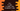# C++ if...else, if..elseif...else and nested if...else## Introduction :

if…else statements are decesion making statements and these statements are mostly used statements in any programming language. In this post, we will learn how to use if…else in c++ with examples.

### C++ if statement :

The syntax of C++ if statement is as below :

``````if(condition){
// run these statements
}``````

Think this statement as if this .. do that.

Here condition is an expression like something is greater-than zero, less than zero etc. The program will check if this condition is true or not. If it finds that it is true, it will run all statements inside the block i.e. all code inside the curly braces.

Again, if it finds that the condition is false, it will ignore all inside the curly braces block.

Let me show you one small example to make it clear :

``````#include<iostream>
using namespace std;

int main()
{
int n = 10;

if (n > 9)
{
cout << "n is greater than 9" << endl;
}
}``````

#### It will print :

``n is greater than 9``

This is because the if block checks if the value of n is more than 9 or not. Since its value is 10, it prints the line that is printed by the cout statement.

Try to change the value of n and check what it returns.

### C++ if…else statement :

if…else adds one more block called else block with if. It’s syntax is as below :

``````if(condition){

}else{

}``````

It will execute the condition and if its value is true, it runs the code inside if block. Similar to the above example. But if the result of condition is false, it runs the code written inside else block. If we change the above example :

``````#include <iostream>
using namespace std;

int main()
{
int n = 1;

if (n > 9)
{
cout << "n is greater than 9" << endl;
}
else
{
cout << "n is less than 9" << endl;
}
}``````

It will execute the else block and print that n is less than 9.

### if…elseif…else :

if…elseif…else adds one more extra block to if…else. It is called elseif. This block runs if if block is not true but else if block is true. It is similar like having two if blocks.

#### Syntax :

``````if(condition){
// if block code
}else if(condition){
// else if block code
}else{
// else block code
}``````

#### Example :

``````#include <iostream>
using namespace std;

int main()
{
int n = 8;

if (n > 9)
{
cout << "n is greater than 9" << endl;
}
else if (n == 8)
{
cout << "n is equal to 8" << endl;
}
else
{
cout << "n is less than 9" << endl;
}
}``````

It will execute the else if block.

### nested if…else :

As the name indicates, we can have one if…else inside another if…else like below :

``````#include <iostream>
using namespace std;

int main()
{
int n = 18;

if (n > 9)
{
if (n % 2 == 0)
{
cout << "n is greater than 9 and even" << endl;
}
else
{
cout << "n is greater than 9 and odd" << endl;
}
}
else
{
cout << "n is less than 9" << endl;
}
}``````

It executes the outer if/else block first and then moves to the inner blocks.